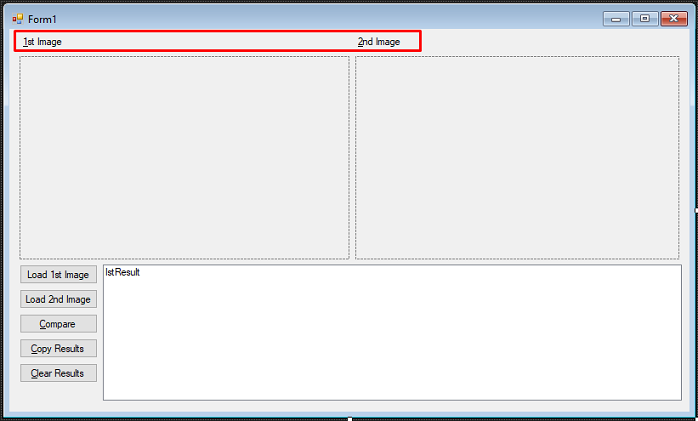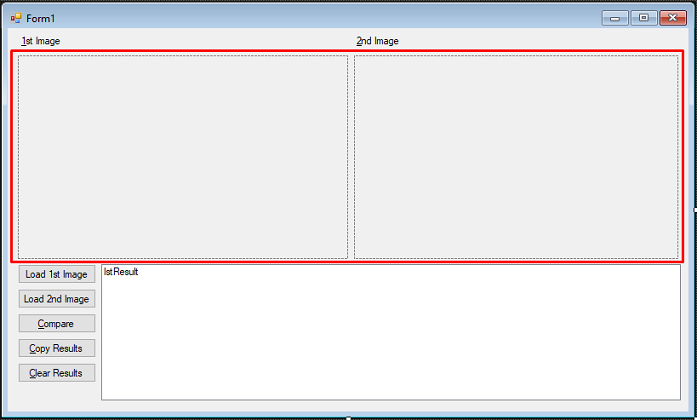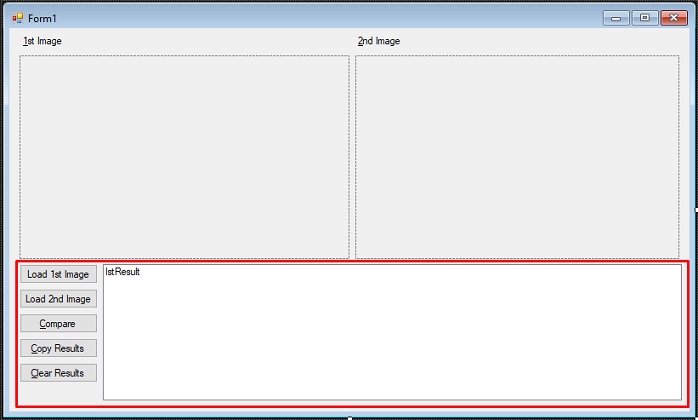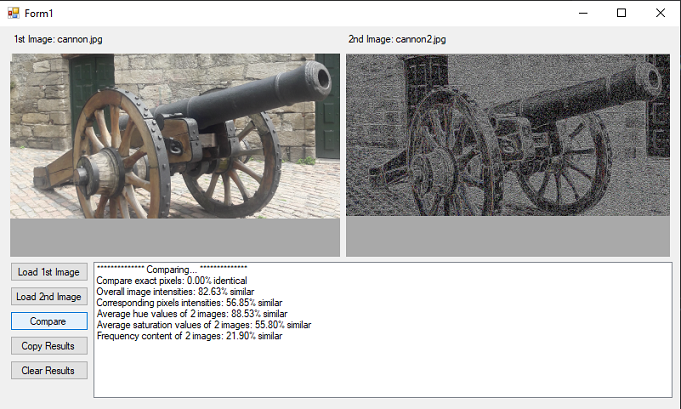﻿ Compare Images with Image Processing - WinForms C# | LEADTOOLS SDK Tutorials Help
In This Topic ▼

# Compare Images with Image Processing - WinForms C#

This tutorial shows how to use image processing techniques to calculate different measures of similarity between two images in a WinForms C# application using the LEADTOOLS SDK.

Overview
Summary This tutorial shows how to compare images using image processing in a WinForms C# application.
Completion Time 30 minutes
Platform Windows WinForms C# Application
IDE Visual Studio 2017, 2019

## Required Knowledge

Get familiar with the basic steps of creating a project by reviewing the Add References and Set a License tutorial, before working on the Compare Images with Image Processing - WinForms C# tutorial.

In Visual Studio, create a new C# Windows Winforms project, and add the below necessary LEADTOOLS references.

The references needed depend upon the purpose of the project. References can be added by one or the other of the following two methods (but not both).

If using NuGet references, this tutorial requires the following NuGet packages:

• `Leadtools.Image.Processing`
• `Leadtools.Viewer.Controls.WinForms`

If using local DLL references, the following DLLs are needed.

The DLLs are located at `<INSTALL_DIR>\LEADTOOLS21\Bin\Dotnet4\x64`:

• `Leadtools.dll`
• `Leadtools.Codecs.dll`
• `Leadtools.Codecs.Cmp.dll`
• `Leadtools.Codecs.Tif.dll`
• `Leadtools.Controls.WinForms.dll`
• `Leadtools.ImageProcessing.Color.dll`
• `Leadtools.ImageProcessing.Core.dll`
• `Leadtools.ImageProcessing.Effects.dll`
• `Leadtools.ImageProcessing.Kernel.dll`

For a complete list of which DLL files are required for your application, refer to Files to be Included With Your Application.

The License unlocks the features needed for the project. It must be set before any toolkit function is called. For details, including tutorials for different platforms, refer to Setting a Runtime License.

There are two types of runtime licenses:

• Evaluation license, obtained at the time the evaluation toolkit is downloaded. It allows the toolkit to be evaluated.
• Deployment license. If a Deployment license file and developer key are needed, refer to Obtaining a License.

Note

## Create the User Interface

With the project created, the references added, and the license set, coding can begin.

In the Solution Explorer, double-click `Form1.cs` to display the `Designer`. Open the Toolbox and add two Labels to the top of `Form1`, as shown in the screenshot below. Leave the names of the new `Labels` as they are. Edit their text to where `label1's` Text property says `&1st Image`, and `label2's` Text says `&2nd Image`.Add two Panels in the middle of the form, as shown below. Keep the default names of the Panels.Add five new Buttons to the lower left side of `Form1`. See the table below for the new Buttons' Name and Text properties.

Name Text
`btnLoadFirst` `Load 1st Image`
`btnLoadSecond` `Load 2nd Image`
`btnCompare` `&Compare`
`btnCopy` `&Copy Results`
`btnClearList` `&Clear Results`

Add a ListBox to the right of the Buttons as shown below. Name the ListBox `lstResult`.## Initialize the Image Viewers and Load the Images

With the UI designed, click on `Form1` in the designer. Go to the `Form1` properties and double-click the `Load` event to create a `Load` event handler for the form. This will bring up the code behind the form. Add the `using` statements below to the top.

C#
````using System; `
`using System.Drawing; `
`using System.IO; `
`using System.Windows.Forms; `
`using Leadtools; `
`using Leadtools.Codecs; `
`using Leadtools.Controls; `
`using Leadtools.ImageProcessing; `
`using Leadtools.ImageProcessing.Color; `
`using Leadtools.ImageProcessing.Core; `
`using Leadtools.ImageProcessing.Effects; ````

Add the following global variables to the `Form1` class.

C#
````private ImageViewer _viewer1; `
`private ImageViewer _viewer2; `
`private RasterCodecs _codecs; `
`private RasterImage _image1; `
`private RasterImage _image2; ````

Add the code below to the `Form1_Load` event handler to initialize the ImageViewer objects.

C#
````private void Form1_Load(object sender, EventArgs e) `
`{ `
`    try `
`    { `
`        _viewer1 = new ImageViewer(); `
`        _viewer1.Dock = DockStyle.Fill; `
`        _viewer1.BackColor = Color.DarkGray; `
`        panel1.Controls.Add(_viewer1); `
`        _viewer1.BringToFront(); `
`        _viewer1.Zoom(ControlSizeMode.Fit, 1.0, _viewer1.DefaultZoomOrigin); `
` `
`        _viewer2 = new ImageViewer(); `
`        _viewer2.Dock = DockStyle.Fill; `
`        _viewer2.BackColor = Color.DarkGray; `
`        panel2.Controls.Add(_viewer2); `
`        _viewer2.BringToFront(); `
`        _viewer2.Zoom(ControlSizeMode.Fit, 1.0, _viewer1.DefaultZoomOrigin); `
` `
`        _codecs = new RasterCodecs(); `
`    } `
`    catch (Exception ex) `
`    { `
`        MessageBox.Show(ex.ToString()); `
`    } `
`} ````

Using the Solution Explorer, navigate back to the `Form1` designer. Double-click on both `Load 1st Image` and `Load 2nd Image` Buttons, to create an event handler for each of the two Buttons and to bring up the code behind the form. Add the code below to the respective event handlers to load the two images to compare.

C#
````private void btnLoadFirst_Click(object sender, EventArgs e) `
`{ `
`    try `
`    { `
`        string _filePath = GetFilePath(); `
`        string _fileName = Path.GetFileName(_filePath); `
`        _image1 = _codecs.Load(_filePath); `
`        _viewer1.Image = _image1; `
`        label1.Text = \$"1st Image: {_fileName}"; `
`    } `
`    catch (Exception ex) `
`    { `
`        MessageBox.Show(ex.ToString()); `
`    } `
`} ````
C#
````private void btnLoadSecond_Click(object sender, EventArgs e) `
`{ `
`    try `
`    { `
`        string _filePath = GetFilePath(); `
`        string _fileName = Path.GetFileName(_filePath); `
`        _image2 = _codecs.Load(_filePath); `
`        _viewer2.Image = _image2; `
`        label2.Text = \$"2nd Image: {_fileName}"; `
`    } `
`    catch (Exception ex) `
`    { `
`        MessageBox.Show(ex.ToString()); `
`    } `
`} ````

Create a new method inside the `Form1` class named `GetFilePath()`. Call this method inside the event handlers shown above. Add the code below to use the OpeFileDialog to select the images you wish to compare.

C#
````private string GetFilePath() `
`{ `
`    string _filePath = null; `
`    OpenFileDialog dlg = new OpenFileDialog(); `
`    dlg.InitialDirectory = @"C:\LEADTOOLS21\Resources\Images"; `
`    if (dlg.ShowDialog(this) == DialogResult.OK) `
`    { `
`        _filePath = dlg.FileName; `
`    } `
`    return _filePath; `
`} ````

## Add the Image Compare Code

Navigate back to the `Form1` designer, using the Solution Explorer. Double-click on the `Compare`, `Copy Results`, and `Clear Results` Buttons to create their event handlers and bring up the code behind the form.

Add the code below to the `btnCompare_Click` event handler to run 5 of the 6 image processing algorithms used in this tutorial for image comparison.

C#
````private void btnCompare_Click(object sender, EventArgs e) `
`{ `
`    if (_viewer1.Image == null || _viewer2.Image == null) `
`    { `
`        MessageBox.Show("Please load images first"); `
`        return; `
`    } `
`    lstResult.Items.Add("************** Comparing... **************"); `
` `
`    int newItem; `
` `
`    // First measure: compare pixel-by-pixel by XOR-ing them `
`    using (RasterImage dstImage = _image2.Clone()) `
`    { `
`        LeadRect rc = new LeadRect(0, 0, _viewer1.Image.Width, _viewer1.Image.Height); `
`        CombineCommand combine = new CombineCommand(_image1, rc, new LeadPoint(0, 0), `
`            CombineCommandFlags.OperationXor); `
`        combine.Run(dstImage); `
`        // Identical pixels will result in black after XOR. Next code will calculate black ratio in resulting image `
`        // Define a region of all black pixels `
`        dstImage.AddColorToRegion(RasterColor.Black, RasterRegionCombineMode.Set); `
`        // Find the area of black region and divide by total image area `
`        double percentBlack = dstImage.CalculateRegionArea() * 100.0 / (dstImage.Width * dstImage.Height); `
`        newItem = lstResult.Items.Add(string.Format("Compare exact pixels: {0:N2}% identical", percentBlack)); `
`        lstResult.TopIndex = newItem; `
`    } `
` `
`    // Make grayscale copies to calculate intensity values `
`    GrayscaleCommand gray = new GrayscaleCommand(8); `
`    // Statistics info command will be used throughout to find average values of image pixels `
`    StatisticsInformationCommand stats = new StatisticsInformationCommand(RasterColorChannel.Master, 0, 255); `
`    using (RasterImage image2Gray = _image2.Clone()) `
`    using (RasterImage image1Gray = _image1.Clone()) `
`    { `
`        LeadRect rc = new LeadRect(0, 0, image2Gray.Width, image2Gray.Height); `
`        // To get intensity values alone, convert to grayscale `
`        gray.Run(image1Gray); `
`        gray.Run(image2Gray); `
` `
`        // Second measure: Overall intensity value `
`        stats.Run(image1Gray); `
`        double intensityAverage1 = stats.Mean; `
`        stats.Run(image2Gray); `
`        double intensityAverage2 = stats.Mean; `
`        double AvgSimilarity = 100.0 - (Math.Abs(intensityAverage2 - intensityAverage1) * 100.0 / 255); `
`        newItem = lstResult.Items.Add(string.Format("Overall image intensities: {0:N2}% similar", AvgSimilarity)); `
`        lstResult.TopIndex = newItem; `
` `
`        // Third measure: pixel-wise intensity similarity  `
`        CombineCommand combine = new CombineCommand(image1Gray, rc, new LeadPoint(0, 0), `
`            CombineCommandFlags.AbsoluteDifference); `
`        combine.Run(image2Gray); `
`        // The image2Gray now has the subtraction result of the 2 image's intensities. `
`        // The Mean value will be the average of the intensities difference `
`        stats.Run(image2Gray); `
`        double pixelsSimilarity = 100.0 - (stats.Mean * 100.0 / 255); `
`        newItem = lstResult.Items.Add(string.Format("Corresponding pixels intensities: {0:N2}% similar", `
`            pixelsSimilarity)); `
`        lstResult.TopIndex = newItem; `
`    } `
` `
`    // Fourth and fifth measures: compare average hue and average saturation `
`    // Color seprate to obtain Hue and Saturation planes `
`    ColorSeparateCommand colorSep = new ColorSeparateCommand(ColorSeparateCommandType.Hsv); `
`    colorSep.Run(_image1); `
`    colorSep.DestinationImage.Page = 1; `
`    stats.Run(colorSep.DestinationImage); `
`    // Store average value of first hue plane `
`    double averageHue1 = stats.Mean; `
`    // Move from the Hue plane to the Saturation plane, which is in page 2 `
`    colorSep.DestinationImage.Page = 2; `
`    stats.Run(colorSep.DestinationImage); `
`    // Store average value of first saturation plane `
`    double averageSat1 = stats.Mean; `
`    // Perform color separation of second image `
`    colorSep.Run(_image2); `
`    colorSep.DestinationImage.Page = 1; `
`    stats.Run(colorSep.DestinationImage); `
`    // Store average value of second image's hue plane `
`    double averageHue2 = stats.Mean; `
`    // Move from the Hue plane to the Saturation plane, which is in page 2 `
`    colorSep.DestinationImage.Page = 2; `
`    stats.Run(colorSep.DestinationImage); `
`    // Store average value of second image's saturation plane `
`    double averageSat2 = stats.Mean; `
`    double HueDiff = Math.Abs(averageHue1 - averageHue2); `
`    // If one hue is very high and the other is very low, they're actually `
`    // Close to each other because 0 lies next to 255 `
`    if (HueDiff > 127) `
`        HueDiff = Math.Abs(HueDiff - 254); `
`    double SatDiff = Math.Abs(averageSat1 - averageSat2); `
`    double hueSimilarity = (255 - HueDiff) * 100 / 255.0; `
`    double satSimilarity = (255 - SatDiff) * 100 / 255.0; `
`    lstResult.Items.Add(string.Format("Average hue values of 2 images: {0:N2}% similar", hueSimilarity)); `
`    newItem = lstResult.Items.Add(string.Format("Average saturation values of 2 images: {0:N2}% similar", `
`        satSimilarity)); `
`    lstResult.TopIndex = newItem; `
`    // 6th measure: fequency content deserves its own function `
`    CompareFrequencyContent(_image1.Clone(), _image2.Clone()); `
`} ````

Add a new method to the `Form1` class named `CompareFrequencyContent(RasterImage img1, RasterImage img2)`. Call this method at the end of the `btnCompare_Click` event handler, as shown above. Add the code below to run the 6th image processing algorithm, which will calculate the frequency content.

C#
````public void CompareFrequencyContent(RasterImage img1, RasterImage img2) `
`{ `
`    // Make sure the images are the same size `
`    if ((img1.Width != img2.Width) || (img1.Height != img2.Height)) `
`    { `
`        SizeCommand sizecommand = new SizeCommand(256, 256, Leadtools.RasterSizeFlags.Bicubic); `
`        sizecommand.Run(img1); `
`        sizecommand.Run(img2); `
`    } `
`    // Use the same flags for both images `
`    // Include the padding flag so that FFT will work with non power-of-2 size images `
`    FastFourierTransformCommandFlags fftFlags = FastFourierTransformCommandFlags.FastFourierTransform `
`        | FastFourierTransformCommandFlags.Gray | FastFourierTransformCommandFlags.PadOptimally; `
`    // The 2 arrays to hold the FFT result `
`    FourierTransformInformation FTArray1 = new FourierTransformInformation(img1, fftFlags); `
`    FourierTransformInformation FTArray2 = new FourierTransformInformation(img2, fftFlags); `
`    // Apply FFT to first image `
`    FastFourierTransformCommand command = new FastFourierTransformCommand(FTArray1, fftFlags); `
`    command.Run(img1); `
`    // Apply FFT to second image `
`    command.FourierTransformInformation = FTArray2; `
`    command.Run(img2); `
`    // Summation of difference between each frequency component magnitude and its counterpart from the other image `
`    double magDiff = 0; `
`    // Summation of the 2 magnitude values from both images for all frequency components `
`    double magSum = 0; `
`    int dataLen = FTArray1.Data.Length; `
`    unsafe // Fastest way to process is to use the data pointer provided by the command `
`    { `
`        double* pData1 = (double*)FTArray1.DataPointer; `
`        double* pData2 = (double*)FTArray2.DataPointer; `
` `
`        for (int i = 0; i < dataLen; ++i) `
`        { `
`            double r1 = *pData1; `
`            ++pData1; `
`            double i1 = *pData1; `
`            ++pData1; `
`            // Magnitude is the square root of the real and imaginary parts `
`            double mag1 = Math.Sqrt(r1 * r1 + i1 * i1); `
`            double r2 = *pData2; `
`            ++pData2; `
`            double i2 = *pData2; `
`            ++pData2; `
`            double mag2 = Math.Sqrt(r2 * r2 + i2 * i2); `
`            // Tally the magnitude sums `
`            magSum += (mag1 + mag2); `
`            // Tally the magnitude differences `
`            magDiff += Math.Abs(mag1 - mag2); `
`        } `
`    } `
`    if (magSum == 0) `
`        magSum = 1; // don't divide by zero `
`    double freqSimilarity = (magSum - magDiff) * 100 / magSum; `
`    int newItem = lstResult.Items.Add(string.Format("Frequency content of 2 images: {0:N2}% similar", freqSimilarity)); `
`    lstResult.TopIndex = newItem; `
`    img1.Dispose(); `
`    img2.Dispose(); `
`} ````

Next, add the code below to the `btnCopy_Click` event handler to copy the results in the ListBox to your clipboard.

C#
````private void btnCopy_Click(object sender, EventArgs e) `
`{ `
`    string listText = ""; `
`    foreach (string txt in lstResult.Items) `
`        listText += txt + Environment.NewLine; `
`    if (listText == "") `
`        return; `
`    Clipboard.Clear(); `
`    Clipboard.SetText(listText); `
`    MessageBox.Show("Results copied to clipboard"); `
`} ````

Lastly, add the code below to the `btnClearList_Click` event handler to clear the results in the ListBox.

C#
````private void btnClearList_Click(object sender, EventArgs e) `
`{ `
`    lstResult.Items.Clear(); `
`} ````

## Run the Project

Run the project by pressing F5, or by selecting Debug -> Start Debugging.

If the steps were followed correctly, the application runs and the form should appear. To test, follow the steps below:

1. Click on Load 1st Image to bring up the OpenFileDialog.

3. Repeat the previous steps, but with the Load 2nd Image button.

4. Once you have the 2 images you wish to compare, click the Compare button.## Wrap-up

This tutorial showed how to compare two images using multiple image processing algorithms.